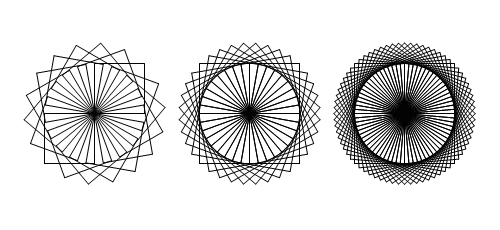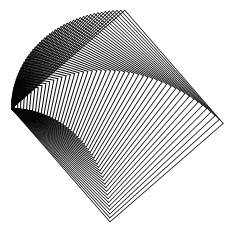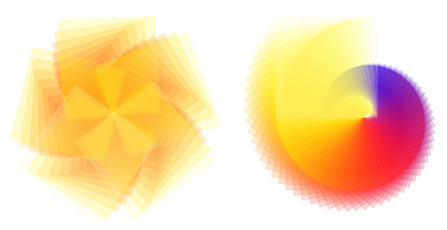## Functions and variables

Splitting of a large algorithm into smaller and simpler tasks is the basic skill of programming. Code portions - functions (and also classes, at more advanced levels), which may be used in a program many times and make it easier to record the whole algorithm, can be prepared for such tasks.
In this chapter we will show you how to program:

### The simplest functions

In order to draw a square, in the previous chapter we used a step forward and turn repeating loop. Many squares can be drawn by placing this loop inside another `repeat` loop:repeat 9 [
repeat 4 [forward 100 right 90]
right 40
wait 100 ;hold the execution for 0.1 second
]

or we can write a square drawing function and place the call to the function in the loop:

to square  ; <--- function square definition
repeat 4 [forward 100 right 90]
end        ; <--- the end of definition

repeat 9 [
square   ; <--- call to the function
right 40
wait 100 ;hold the execution for 0.1 second
]

Both program versions will work the same way, but the loop code with a function is more clear, which is very important in programming. Any other part of the program will also be able to use the square function, and if there is a need to change the way of drawing of all squares in the program (for example, change of colour) - this can be done in one place only: in the `square` code.
The `wait` command stops the program for a specified number of milliseconds (1 seconds = 1000 milliseconds). This will help to see the progress of drawing squares in the loop.

### Variables

Variables allow to store the values (numbers, strings, charts...) during the program execution. LOGO variables is a very broad subject and now we will present only the nutshell that is necessary for further work with functions.
The LOGO variables (and the POOL ones at the same time) have three important features:

• variables can be created in any part of a program code;
• a single variable can store different types of data (we call it is of a dynamic type of data), e.g. text can be assigned to a variable that initially stored a number;
• LOGO tries to match a variable type (or the whole expression) to the type expected in a given expression, e.g. adding number 5 and the text that reads "5" is correct and the result is number 10.

To create variables, we will use the `make` command now. Its arguments are: variable name and its initial value. The same command is used to assign a new value to a variable that already exists.

Important information:

• the name of a variable is a word, that is a string of characters; in LOGO, a string begins with a quotation mark, e.g.: `"name`
• the value of a variable can be read with the use of the instruction `thing "name`; because reading of variables is a very frequent operation, the `thing "name` has its abbreviation: `:name` (note that the name here doesn’t have a quotation mark);
• in POOL we can use a more natural syntax: an equivalent of `make "name value` is `"name := value`

A few lines of the program that illustrates operations on variables are presented below:

make "x 1      ;1) variable "x" with the value of 1 is created
print :x       ;2) prints out the value of "x"

make "y :x + 1 ;3) variable "y" with the value of 2 is created
make "x 2 * :y ;4) the new value, 4, is assigned to "x"
(print :x :y)  ;5) prints out values of variables "x" and "y"

"z := :x + :y  ;6) consise syntax used in POOL
print :z

make "x "10      ;7) the new value of "x" is now the word "10"
(print "word :x;8) prints out: "word 10"
print 2 * :x     ;9) prints out the result of the multiplication: 20

The result of the program execution (in the text window):

1
4 2
6
word 10
20

In the square drawing program we can add the `:n` variable to change the number of squares easily:to square
repeat 4 [forward 50 right 90]
end

make "n 20 ;try also: 40, 10, 5
repeat round 360 / :n [
square
right :n
]

### Functions with arguments

Our function always draws a square with a side length 100, which limits its usability. However, it can be improved and the length of a side can be passed to a function as an argument. In the same way as, for example, a step length in the `forward 100` command, or an angle value in the `right 90` turn has been presented before. A function argument should be defined in its definition. In the function itself, we treat the argument as a variable:

to square :a ; <--- definition of the function with the argument
repeat 4 [forward :a right 90]
print :a    ; <--- print out the value of the argument
end

; calls to the function with the argument:
square 50
square 100
square 150

;print :a  ; <--- this instruction causes an error

Each function call in the program draws a square with a defined side length. This function writes also an argument value in the text window.
Note: the last commented line uses the same command as the function code: `print :a`. Uncommented line (first `;` mark deleted) will cause a runtime error - check it out. A function argument is a local variable, it means that it is available only in the function for which it has been defined.

The program below uses the `square` function with a variable length side. Please, note that the argument in the function call is a result of the expression: `3 * repcount`. The `repcount` command is often used in LOGO loops - it returns the number of the current loop iteration.to square :a ; <--- definition of the function with the argument
repeat 4 [forward :a right 90]
end

repeat 50 [
square 3 * repcount ; <--- call to the function
right 1
]

#### More arguments

A function can have many arguments, it’s easy: in a function definition you have to add next arguments. What is more, functions and commands can have optional arguments, e.g. the `print`, command, which writes argument values in the text window, uses one argument by default, e.g.:

print "ok

but it can also be used with many arguments which will be written in one line in the text window. In order to do so, put the command and its arguments in parentheses, e.g.:

(print 1 "ok 2+3)

Alike commands, also functions can have optional arguments. If we want to pass an optional argument value in the function call, the whole call should be in parentheses. If the value of optional argument is not presented in the function call, the function will ascribe a default value to it.
An optional argument is placed in the function definition together with its default value in square brackets:

to function :arg_required [:arg_opcional value]

The following program defines the `square`, function which has two obligatory arguments `:a` and `:color` and the `:p` optional argument with a default value of `100`.to square :a :color [:p 100] ;draws a filled square:
(setpc :color :p)          ;     a - side length
setps :a                   ; color - fill color
forward :a                 ;     p - opacity (100% by default)
end

hideturtle      ;turtle symbol is hidden
setpalette "hot ;use the color palette named "hot"

make "n 5
make "l 10 ;initial size of the square
make "m 1 + round 360 / :n

repeat :m [
make "k 1000 * repcount / :m ;calculate the index of color

;square :l :k               ; <--- here :p will be 100% by default
(square :l :k 20)           ; <--- here :p is set to 20%

pu bk :l - 4 pd   ;move back the turtle with the pen up
make "l :l + 1.7  ;calculate the square size
right :n   ;try this line...
;right :m  ;...or this line instead the above one
]

### Functions that return result

Functions defined by the programmer can yield a result, alike the LOGO commands, such as the loop iteration counter, `repcount`, or the square root of the number `sqrt x`. Here is an example:

;...............drawing configuration..................
:pool_cfg,"scale_x := 30 ;horizontal scale
:pool_cfg,"scale_y := 30 ;vertical scale
window                   ;turtle can move out of the window
hideturtle               ;turtle symbol is hidden
;......................................................

to pitagoras :a :b         ;right-angled triangle
let "p pos               ;local variable saves the turtle's position
left 90 forward :a
right 90 forward :b
setpos :p                ;go back to the initial position
output sqrt :a^2 + :b^2  ;result: length of the hypotenuse
end

print sqrt 3 * 3 + 4 * 4  ;calculate step by step...
print pitagoras 3 4       ;...or with the function

The program defines a function with two arguments: `:a` and `:b`. This function draws a right-angled triangle with leg length equal to the argument values. The result of this function is a number defining the length of the triangle’s hypotenuse - the `output` command allows to pass the result value to the expression that calls the function. In this case `output` is the last command of the function, but it can also be placed inside the function. It will result in stopping execution of the function (just the same as the `stop` command).
Note, that the function remembers the initial position of the turtle in the `:p` variable, in order to return to the position, having drawn the legs, to draw the hypotenuse. The `:p` variable is a local variable and, similar to the `:a` and `:b` arguments, it is available only inside the function. In POOL, the `let` command allows to create a local variable and ascribe a value to it. In many LOGO dialects this command is called a `localmake` - POOL accepts also this name.
The fragment of the program described as "drawing parameters" includes a code defining the size of turtle’s steps (in screen pixels) and the way of drawing.

### Ending

The ability of programming the functions will be very helpful in further chapters. We will show how to write a recursive function - that is a function which calls itself in its code. We will also present how to write a program in which many turtle-objects operate at the same time, and how to use functions to describe their behavior.